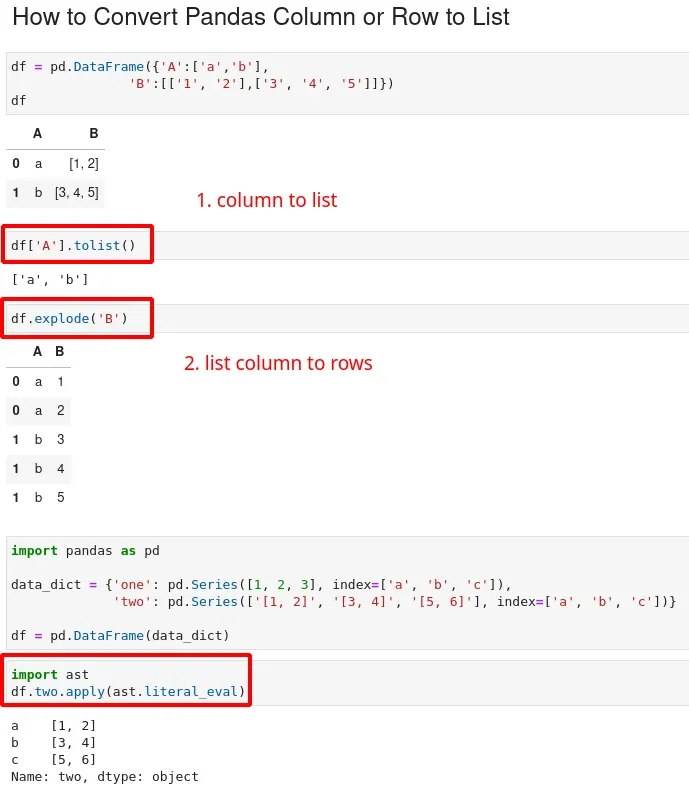To convert a DataFrame column or row to a list in Pandas, we can use the Series method `tolist()`. Here's how to do it:

``````df['A'].tolist()
df.B.tolist()
``````## Setup

We will use the following DataFrame to convert rows and columns to list:

`````````python
import pandas as pd

df = pd.DataFrame({'A': [1, 2, 3], 'B': [4, 5, 6]})
``````

data:

A B
0 1 4
1 2 5
2 3 6

## Convert column to list

To convert a column to a list, we can access the column using either

• the bracket notation `([])`
• or the dot notation `(.)`

and then call the `tolist()` method on the resulting pandas Series object:

``````df['A'].tolist()
df.B.tolist()
``````

result:

``````[1, 2, 3]
[4, 5, 6]
``````

## Convert row to list

To get a list from a row in a Pandas DataFrame, we can use the `iloc` indexer to access the row by its index. Then call the `tolist()` method on the resulting pandas Series object:

``````row_list = df.iloc.tolist()
``````

result:

``````[1, 4]
``````

## Convert column with list values to row

We can convert columns which have list values to rows by using method `.explode()`. The example below shows how to convert column B which has list values. We will use new DataFrame:

``````df = pd.DataFrame({'A':['a','b'],
'B':[['1', '2'],['3', '4', '5']]})
``````

with data:

A B
0 a [1, 2]
1 b [3, 4, 5]

To rows by:

``````df.explode('B')
``````
A B
0 a 1
0 a 2
1 b 3
1 b 4
1 b 5

## Column from lists (string) to lists

Finally let's check how to convert column which contains list values stored as strings to list:

``````import pandas as pd

data_dict = {'one': pd.Series([1, 2, 3], index=['a', 'b', 'c']),
'two': pd.Series(['[1, 2]', '[3, 4]', '[5, 6]'], index=['a', 'b', 'c'])}

df = pd.DataFrame(data_dict)
``````

data looks like:

one two
a 1 [1, 2]
b 2 [3, 4]
c 3 [5, 6]

We can extract list values by using list comprehensions:

``````[x.strip('[]').split(',') for x in df['two']]
``````

which result into new list of lists:

``````[['1', ' 2'], ['3', ' 4'], ['5', ' 6']]
``````

If we like to keep the list values in the column we can use `ast` module:

``````import ast
df.two.apply(ast.literal_eval)
``````

Which we result into:

``````a	[1, 2]
b	[3, 4]
c	[5, 6]
Name: two, dtype: object
``````